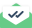# Distance from COM

Dear all,

I want to calculate the distance of each atom in a group from the center of mass of that group. how could I do that?

It should be mentioned that instead of “wrapped” coordinates (x,y,z), we should use “unwrapped” coordinates (xu,yu,zu) which are not accessible in variable command. This is important because when a group of atoms is passing through a PBC, it seems that the group is divided into two separate parts. The distances calculated by “wrapped” coordinates for the atoms in the part including com are correct but for the other part are bigger than the real values (as the com is near one side of the simulation box while those atoms are near the opposite side).

RegardsSent with Mailtrack

Dear all,

I want to calculate the distance of each atom in a group from the center of
mass of that group. how could I do that?

the most obvious direct approach would be to write a custom compute
style for that purpose.

axel.

Dear all,

I want to calculate the distance of each atom in a group from the center of
mass of that group. how could I do that?

It should be mentioned that instead of "wrapped" coordinates (x,y,z), we
should use "unwrapped" coordinates (xu,yu,zu) which are not accessible in
variable command. This is important because when a group of atoms is passing

i forgot to mention, xu,yu,zu _should_ be available in variable
expressions via compute property/atom.

axel.

Thank you dear Axel

It is a big relief that I can finally access xu,yu,zu via compute property/atom. I have tried so many complicated tricks but forgot this simple option.

RegardsSent with Mailtrack

I think you can do this w/out writing a new compute (albeit less efficiently)

by simply using per-atom variables, something like this for group foo:

compute prop foo property/atom xu yu zu

variable dx atom c_prop-xcm(foo,1)
variable dy atom c_prop-xcm(foo,2)
variable dz atom c_prop-xcm(foo,3)

variable dist atom sqrt(dxdx+dydy+dz*dz)

dump 1 foo custom 100 tmp.dump id v_dx v_dy v_dz v_dist

Since “dist” is a per-atom variable (defined for all atoms, not just

those in the group), you can do whatever you want with it.

E.g. dump it out, reduce it, time average it, etc.

As a side note,

if we added a compute that converted a per-chunk

quantity (like COM) into a per-atom quantity by assigning the

per-chunk value to every atom in the chunk … then you could

use similar logic as above to dump out the distance of every atom from the COM of the

chunk it is in. Something like:

compute ID group global/atom c_gID/v_gID glob1 glob2 …

c_gID or v_gID = compute or variable that stores an index for each atom

e.g. the output of compute chunk/atom

glob1, glob2 = references to computes/fixes that store a global quantity

e.g. compute com/chunk

The compute would produce a per-atom vector/array where each column

had the global quantity indexed by the 1st arg.

Steve

Steve

Thank you dear Steve

Your explanations are very helpful. Especially, the way you defined the compute and variables is really concise.

Best RegardsSent with MailtrackSent with Mailtrack